# Creating BO Security Matrix using SBOPRepositoryExplorer

This post contains how we can create an useful security matrix in SAP BO BI using the SBOPRepositoryExplorer connector.

Shortly I want to show you next content for this example of security matrix:

• Access Levels (ACLs) definition;
• Groups and Users Relation;
• Application Rights;
• Folder Rights;
• Universe Rights;
• Connection Rights.

1. How to extract ACLs

Using the universe provided in SBOPRepositoryExplorer and WebIntelligence: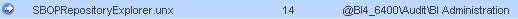We could use next dimensions to extract the ACLs from our CMS repository:

Next step is create some required variables for our example:

=If ([Specific Right]=0 And [Right Group Name]<>�?General�?)

Then “Overwrite General�?

Else

If ([Specific Right]=1 And [Right Group Name]<>�?General�?)

Then “Specific Right�?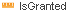=If  (Count([CRole Right ID]) Where([CRole Right Granted]=1))>0

Then 1

Else

If (Count([CRole Right ID]) Where([CRole Right Granted]=0))>0

Then 0

Else Count([CRole Right ID])

Now we can create a cross-table like:

And for the values () we can use conditional formatting rules:

And the final result is (example):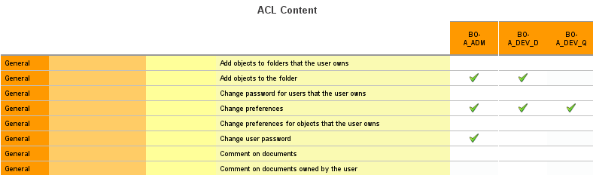2. Groups and Users Relation

For thiskind of content we can use different perspectives/views in function of our requirements, but anyway, we can use next basic dimensions: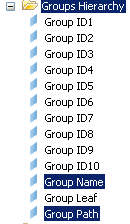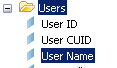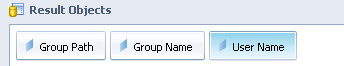Here we have an example with:

“Group Name�?

“User Name�?

and for the value we can define next formula:

=If Count([Group Name])>=1

Then “X�?

Else “�?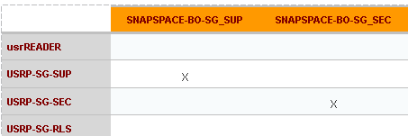Other example using full path:

3. Application Rights

For applications we can use next dimensions: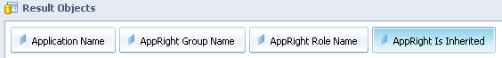A possible example: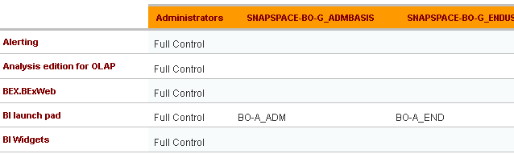4. Folder Rights

For folder rights we can use the same logic than application rights:

5. Universe Rights

In next example we are showing rights for universe folders:

6. Connection Rights

Like the previous one we can use next dimensions: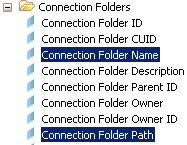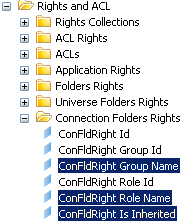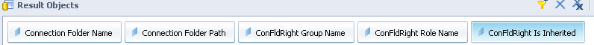This is a simple way to create online our security matrix for SAP BO BI.

` `

Thanks and enjoy!

Jorge Sousa